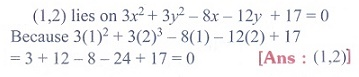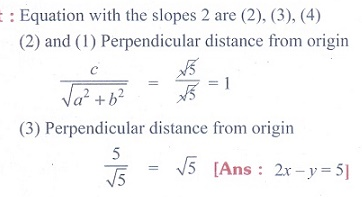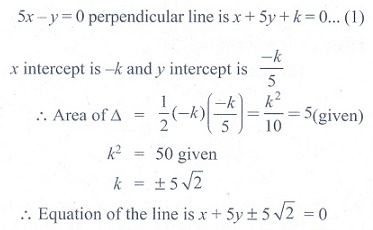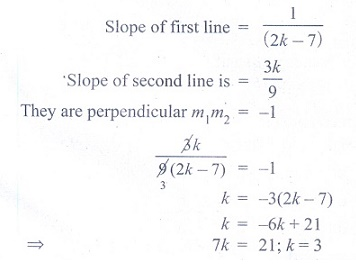Home | | Maths 11th std | Exercise 6.5: Choose the correct answer

# Exercise 6.5: Choose the correct answer

Multiple choice questions with answers / choose the correct answer with answers - Maths Book back 1 mark questions and answers with solution for Exercise Problems - Mathematics : Two Dimensional Analytical Geometry

CHAPTER : Two Dimensional Analytical Geometry

Choose the correct or more suitable answer

1. The equation of the locus of the point whose distance from y-axis is half the distance from origin is

(1) x2 + 3y2 = 0

(2) x2 - 3y2 = 0

(3) 3x2 + y2 = 0

(4) 3x2 - y2 = 0

Solution2. Which of the following equation is the locus of (at2, 2at)(3) x2 + y2 = a2

(4) y2 = 4ax

Solution3. Which of the following point lie on the locus of 3x2 + 3y2 - 8x - 12y + 17 = 0

(1) (0, 0)

(2) (-2, 3)

(3) (1, 2)

(4) (0,-1)

Solution4. If the point (8,-5) lies on the locus x2/16 - y2/25 = k, then the value of k is

(1) 0

(2) 1

(3) 2

(4) 3

Solution5. Straight line joining the points (2, 3) and (-1, 4) passes through the point (α,β) if

(1) α + 2β =7

(2) 3α + β =9

(3) α + 3β =11

(4) 3α + β =11

Solution6. The slope of the line which makes an angle 45̊ with the line 3x - y = -5 are

(1) 1, -1

(2) 1/2, -2

(3) 1,1/2

(4) 2, -1/2

Solution7. Equation of the straight line that forms an isosceles triangle with coordinate axes in the I-quadrant with perimeter 4 + 2√2 is

(1) x + y + 2 = 0

(2) x + y - 2 = 0

(3) x + y - √2 = 0

(4) x + y + √2 = 0

Solution8. The coordinates of the four vertices of a quadrilateral are (-2,4), (-1,2), (1,2) and (2,4) taken in order.The equation of the line passing through the vertex (-1,2) and dividing the quadrilateral in the equal areas is

(1) x+ 1 = 0

(2) x + y = 1

(3) x + y + 3 = 0

(4) x - y + 3 = 0

Solution9. The intercepts of the perpendicular bisector of the line segment joining (1, 2) and (3,4) with

coordinate axes are

(1) 5, -5

(2) 5, 5

(3) 5, 3

(4) 5, -4

Solution10. The equation of the line with slope 2 and the length of the perpendicular from the origin equal to √5 is

(1) x + 2y = √5

(2) 2x + y = √5

(3) 2x + y = 5

(4) x + 2y - 5 = 0

Solution11. A line perpendicular to the line 5x - y = 0 forms a triangle with the coordinate axes. If the area of the triangle is 5 sq. units, then its equation is

(1) x+ 5y ± 5√2 = 0

(2) x- 5y ± 5√2 = 0

(3) 5x+y± 5√2 = 0

(4) 5x-y±5√2 = 0

Solution12. Equation of the straight line perpendicular to the linex-y+5 = 0, through the point of intersection the y-axis and the given line

(1) x - y - 5 = 0

(2) x + y - 5 = 0

(3) x + y + 5 = 0

(4) x + y + 10 = 0

Solution13. If the equation of the base opposite to the vertex (2, 3) of an equilateral triangle is x + y = 2, then the length of a side is

(1) √ 3/2

(2) 6

(3) √6

(4) 3√2

Solution14. The line (p + 2q)x + (p - 3q)y = p - q for different values of p and q passes through the point

(1) (3/2, 5/2)

(2) (2/5, 2/5)

(3) (3/5, 3/5)

(4) (2/5, 3/5)

Solution15. The point on the line 2x - 3y = 5 is equidistance from (1,2) and (3, 4) is

(1) (7, 3)

(2) (4, 1)

(3) (1,-1)

(4) (-2, 3)

Solution16. The image of the point (2, 3) in the line y = -x is

(1) (-3, -2)

(2) ( -3, 2 )

(3) ( -2, -3)

(4) ( 3, 2 )

Solution17. The length of  ┴ from the origin to the line x/3 y/4 = 1, is

(1) 11/5

(2) 5/12

(3) 12/5

(4) -5/12

Solution18. The y-intercept of the straight line passing through (1,3) and perpendicular to 2x - 3y + 1 = 0 is

(1) 3/2

(2) 9/2

(3) 2/3

(4) 2/9

Solution19. If the two straight lines x + (2k - 7)y + 3 = 0 and 3kx + 9y - 5 = 0 are perpendicular then the

value of k is

(1) k = 3

(2) k = 1/3

(3) k = 2/3

(4) k = 3/2

Solution20. If a vertex of a square is at the origin and its one side lies along the line 4x + 3y - 20 = 0, then the area of the square is

(1) 20 sq. units

(2) 16 sq. units

(3) 25 sq. units

(4) 4 sq.units

Solution21. If the lines represented by the equation 6x2 + 41xy - 7y2 = 0 make angles
and  with x- axis then tanα tanβ =

(1) -6/7

(2) 6/7

(3) -7/6

(4) 7/6

Solution22. The area of the triangle formed by the lines x2 - 4y2 = 0 and x = a is

(1) 2a2

(2) √3/2 a2

(3) 1/2 a2

(4) 2/√3 a2

Solution23. If one of the lines given by 6x2 - xy + 4cy2 = 0 is 3x + 4y = 0,, then c equals to

(1) -3

(2) -1

(3) 3

(4) 1

Solution24. θ is acute angle between the lines x2 - xy - 6y2 = 0, thenis

(1) 1

(2) -1/9

(3) 5/9

(4) 1/9

Solution25. The equation of one the line represented by the equation x2 + 2xy cotθ - y2 = 0 is

(1) x - y cot θ = 0

(2) x + y tan θ = 0

(3) x cos θ + y (sinθ + 1) = 0

(4) x sin θ + y (cosθ + 1) = 0

SolutionTags : Two Dimensional Analytical Geometry | Mathematics , 11th Mathematics : UNIT 6 : Two Dimensional Analytical Geometry
Study Material, Lecturing Notes, Assignment, Reference, Wiki description explanation, brief detail
11th Mathematics : UNIT 6 : Two Dimensional Analytical Geometry : Exercise 6.5: Choose the correct answer | Two Dimensional Analytical Geometry | Mathematics